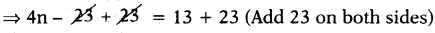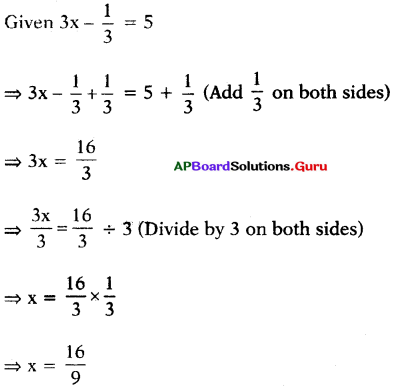# AP Board 7th Class Maths Solutions Chapter 3 Simple Equations Ex 3.2

SCERT AP 7th Class Maths Solutions Pdf Chapter 3 Simple Equations Ex 3.2 Textbook Exercise Questions and Answers.

## AP State Syllabus 7th Class Maths Solutions 3rd Lesson Simple Equations Exercise 3.2

Question 1.
Give the steps you will use to separate the variables and then solve the equation.
(i) $$\frac{5 m}{3}$$ = 10
Given $$\frac{5 m}{3}$$ = 10
⇒ $$\frac{5 m}{3}$$ × 3 = 10 × 3 (Multiply with 3 on both sides)
⇒ 5m = 30
⇒ $$\frac{5 m}{5}$$ = $$\frac{30}{5}$$ (Divide both sides by 5)
⇒ m = 6

Check:
Substitute m = 6 in the given equation,
LHS = $$\frac{5 m}{3}$$ = $$\frac{5(6)}{3}$$ = $$\frac{30}{3}$$ = 10 = RHS.
Hence verified.(ii) 4M – 23 = 13
Given 4n – 23 = 13⇒ 4n = 36
⇒ $$\frac{4 n}{4}$$ = $$\frac{36}{4}$$ (Divide both sides by 4)
⇒ n = 9

Check:
Substitute n = 9 in the given equation.
LHS = 4n – 23
= 4(9) – 23
= 36 – 23 = 13 = RHS
Hence verified.

(iii) – 5 + 3x = 16
Given – 5 + 3x = 16
⇒ – 5 + 3x + 5 = 16 + 5 (Add 5 on both sides)
⇒ 3x = 21
⇒ $$\frac{3 x}{3}$$ = $$\frac{21}{3}$$ (Divide by 3 on both sides)
⇒ x = 7

Check:
Substitute x = 7 in the given equation
LHS = – 5 + 3x
= – 5 + 3(7)
= – 5 + 21 = 16 = RHS
Hence verified.(iv) 2(y – 1) =8
Given 2(y – 1) = 8
⇒ 2y – 2 = 8 (Distributive property)
⇒ 2y – 2 + 2 = 8 + 2 (Add 2 on both sides)
⇒ 2y = 10
⇒ $$\frac{2 y}{2}$$ = $$\frac{10}{2}$$ (Divide by 2 on both sides)
⇒ y = 5

Check: Substitute y = 5 in the given equation
LHS = 2(y – 1)
= 2(5 – 1)
= 2 × 4 = 8 = RHS
Hence verified.

Question 2.
Solve the following simple equations and check the results.
(i) 3x = 18
Given 3x = 18
⇒ $$\frac{3 x}{3}$$ = $$\frac{18}{3}$$ (Divide by 3 on both sides)
⇒ x = 6

Check: Substitute x = 6 in 3x = 18
LHS ⇒ 3x = 3(6) = 18 = RHS
Hence verified.

(ii) $$\frac{b}{7}$$ = – 2
Sol.
Given $$\frac{b}{7}$$ = – 2
⇒ $$\frac{b}{7}$$ × 7 = – 2 × 7 (Multiply by 7 on both sides)
⇒ b = – 14

Check: Substitute b = – 14 in
$$\frac{b}{7}$$ = – 2
LHS = $$\frac{b}{7}$$ = $$\frac{- 14}{7}$$ = – 2 = RHS
Hence verified(iii) – 2x = – 10
Given – 2x = – 10
⇒ $$\frac{-2 x}{-2}$$ = $$\frac{-10}{-2}$$ (Divide by – 2 on both sides)
⇒ x = 5

Check:
Substitute x = 5 in
– 2x = – 10
LHS = – 2x
= – 2(5) = – 10 = RHS
Hence verified.

(iv) 10 + 6a = 40
Given 10 + 6a = 40
⇒ 10 + 6a – 10 = 40 – 10 (Subtract 10 on both sides)
⇒ 6a = 30
⇒ $$\frac{6a}{6}$$ = $$\frac{30}{6}$$ (Divide by 6 on both sides)
⇒ a = 5

Check: Substitute a = 5 in 10 + 6a = 40
LHS = 10 + 6a
= 10 + 6(5)
= 10 + 30
= 40 = RHS
Hence verified.

(v) – 7m = 21
Given – 7m = 21
⇒ $$\frac{-7 m}{-7}$$ = $$\frac{21}{-7}$$ (Divide by – 7 on both sides)
⇒ m = – 3

Check:
Substitute m = in – 7m = 21
LHS = – 7m
= – 7 (- 3)
= 21 = RHS
Hence verified.(iv) 4p + 7 = – 21
Given 4p + 7 = – 21
⇒ 4p + 7 – 7 = – 21 – 7 (Subtract 7 on both sides)
⇒ 4p = – 28
⇒ $$\frac{4p}{4}$$ = $$\frac{-28}{4}$$ (Divide by 4 on both sides)
⇒ p = – 7

Check:
Substitute p = – 7 in 4p + 7 = – 21
LHS = 4p + 7
= 4(- 7) + 7
= – 28 + 7
= – 21 = RHS
Hence verified.

(vii) 3x – $$\frac{1}{3}$$ = 5Check: Substitute x = $$\frac{16}{9}$$ in 3x – $$\frac{1}{3}$$ = 5
LHS = 3x – $$\frac{1}{3}$$
= 3$$\left(\frac{16}{9}\right)$$ – $$\frac{1}{3}$$
= $$\frac{16}{3}-\frac{1}{3}$$
= $$\frac{16-1}{3}$$ = $$\frac{15}{3}$$
= 5 = RHS
Hence verified.

(viii) 18 – 7n = – 3
Given 18 – 7n = – 3
⇒ 18 – 7n – 18 = – 3 – 18 (Subtract 18 on both sides)
⇒ – 7n = – 21
⇒ $$\frac{-7 n}{-7 n}$$ = $$\frac{-21}{-7}$$ (Divide by – 7 on both sides)
⇒ n = 3

Check:
Substitute n = 3 in 18 – 7n = – 3
LHS = 18 – 7n
= 18 – 7(3)
= 18 – 21
= – 3 = RHS
Hence verified.(ix) 3(k + 4) = 21
Sol.
Given 3(k + 4) = 21
⇒ 3k + 12 = 21 (Distributive property)
⇒ 3k + 12 – 12 = 21 – 12 (Subtract 12 on both sides)
⇒ 3k = 9
⇒ $$\frac{3 \mathrm{k}}{3}$$ = $$\frac{9}{3}$$ (Divide by 3 on both sides)
⇒ k = 3

Check:
Substitute k = 3 in 3(k + 4) = 21
LHS = 3(k + 4)
= 3(3 + 4)
= 3 × 7 = 21= RHS
Hence verified.

(x) 9 (a + 1) + 2 = 11
Given 9(a + 1) + 2 = 11
⇒ 9(a + 1) + 2 – 2 = 11 – 2 (Subtract 2 on both sides)
⇒ 9(a + 1) = 9
⇒ $$\frac{9(a+1)}{9}$$ = $$\frac{9}{9}$$ (Divide by 9 on both sides)
⇒ a + 1 = 1
⇒ a + 1 – 1 = 1 – 1 (Subtract 1 on both sides)
⇒ a = 0

Check:
Substitute a = 0 in 9(a + 1) + 2 = 11
LHS = 9(a + 1) + 2
= 9(0 + 1) + 2
= 9(1) + 2
= 9 + 2 = 11 = RHS
Hence verified.